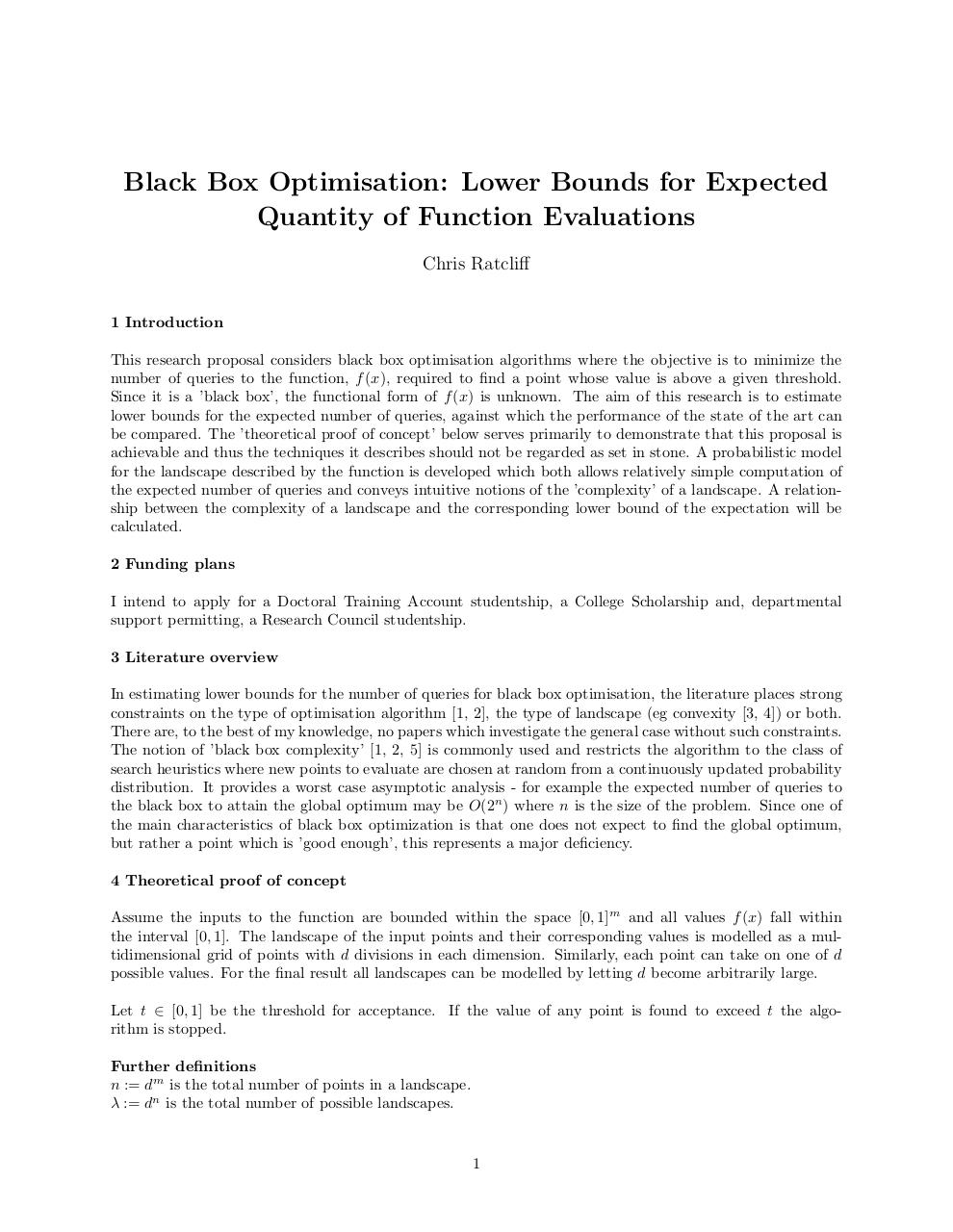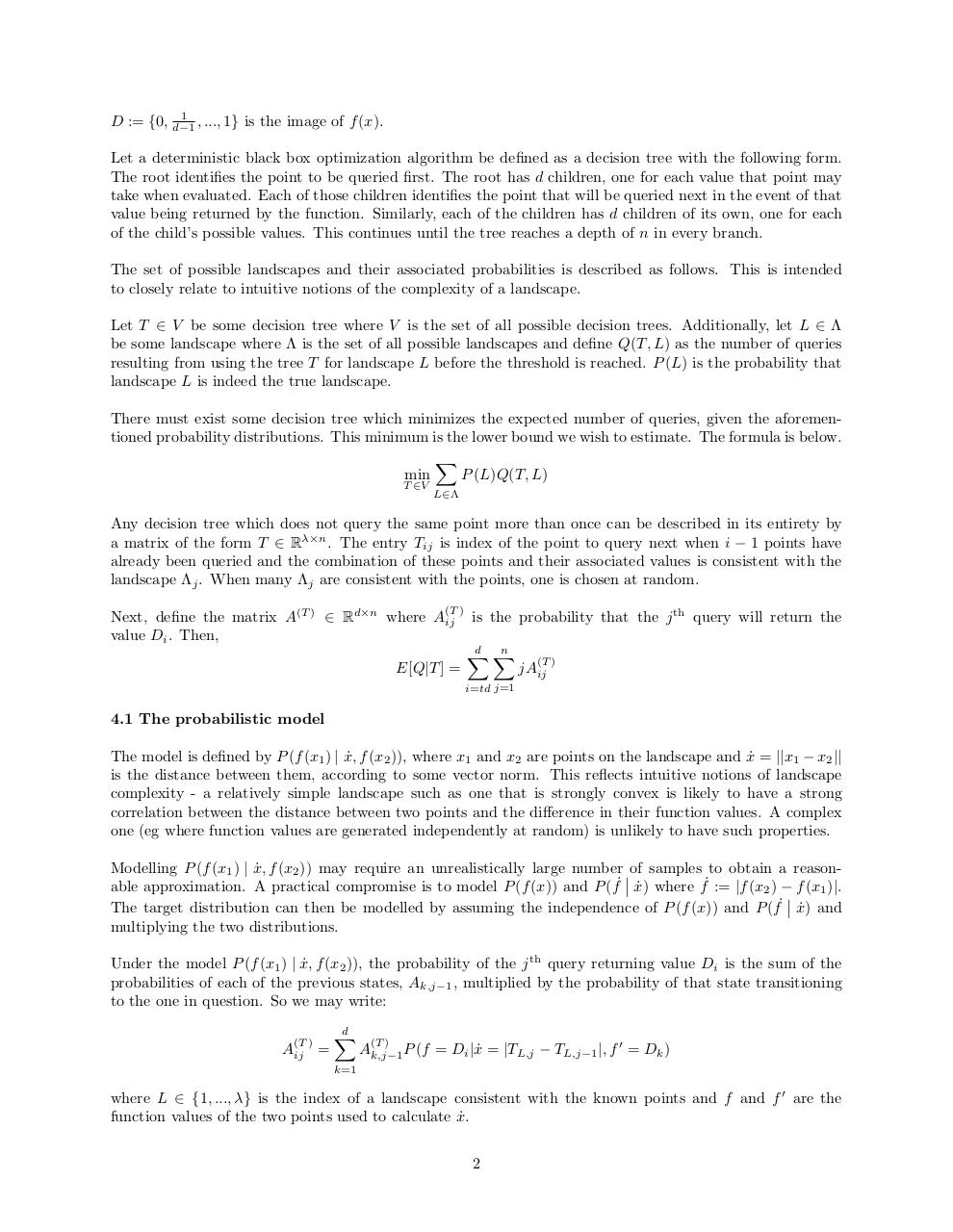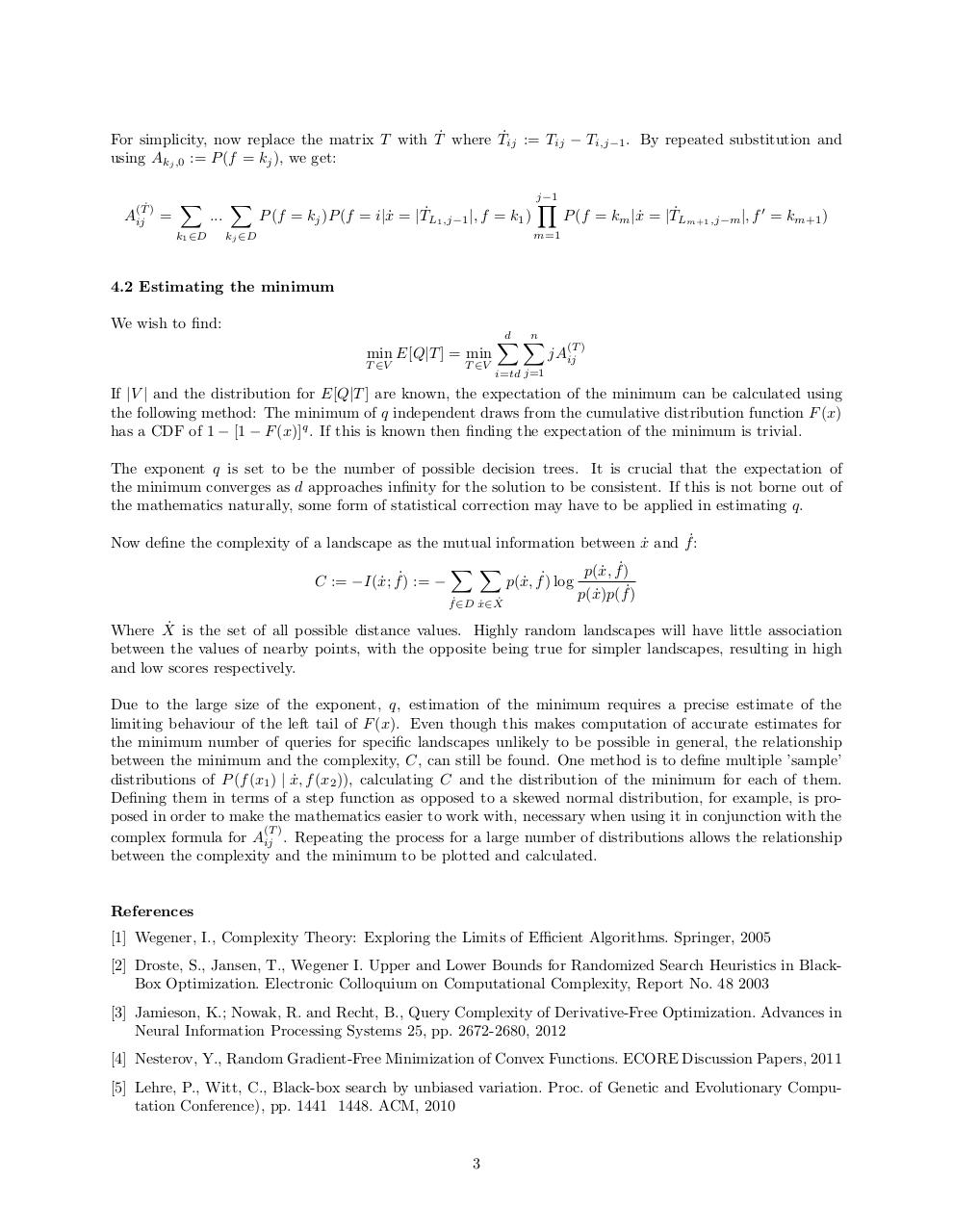# research statement .pdf

### File information

Original filename: research_statement.pdf

This PDF 1.5 document has been generated by TeX / MiKTeX pdfTeX-1.40.14, and has been sent on pdf-archive.com on 09/03/2015 at 21:19, from IP address 86.18.x.x. The current document download page has been viewed 1029 times.
File size: 152 KB (3 pages).
Privacy: public file

research_statement.pdf (PDF, 152 KB)

### Document preview

Black Box Optimisation: Lower Bounds for Expected
Quantity of Function Evaluations
Chris Ratcliff
1 Introduction
This research proposal considers black box optimisation algorithms where the objective is to minimize the
number of queries to the function, f (x), required to find a point whose value is above a given threshold.
Since it is a ’black box’, the functional form of f (x) is unknown. The aim of this research is to estimate
lower bounds for the expected number of queries, against which the performance of the state of the art can
be compared. The ’theoretical proof of concept’ below serves primarily to demonstrate that this proposal is
achievable and thus the techniques it describes should not be regarded as set in stone. A probabilistic model
for the landscape described by the function is developed which both allows relatively simple computation of
the expected number of queries and conveys intuitive notions of the ’complexity’ of a landscape. A relationship between the complexity of a landscape and the corresponding lower bound of the expectation will be
calculated.
2 Funding plans
I intend to apply for a Doctoral Training Account studentship, a College Scholarship and, departmental
support permitting, a Research Council studentship.
3 Literature overview
In estimating lower bounds for the number of queries for black box optimisation, the literature places strong
constraints on the type of optimisation algorithm [1, 2], the type of landscape (eg convexity [3, 4]) or both.
There are, to the best of my knowledge, no papers which investigate the general case without such constraints.
The notion of ’black box complexity’ [1, 2, 5] is commonly used and restricts the algorithm to the class of
search heuristics where new points to evaluate are chosen at random from a continuously updated probability
distribution. It provides a worst case asymptotic analysis - for example the expected number of queries to
the black box to attain the global optimum may be O(2n ) where n is the size of the problem. Since one of
the main characteristics of black box optimization is that one does not expect to find the global optimum,
but rather a point which is ’good enough’, this represents a major deficiency.
4 Theoretical proof of concept
Assume the inputs to the function are bounded within the space [0, 1]m and all values f (x) fall within
the interval [0, 1]. The landscape of the input points and their corresponding values is modelled as a multidimensional grid of points with d divisions in each dimension. Similarly, each point can take on one of d
possible values. For the final result all landscapes can be modelled by letting d become arbitrarily large.
Let t ∈ [0, 1] be the threshold for acceptance. If the value of any point is found to exceed t the algorithm is stopped.
Further definitions
n := dm is the total number of points in a landscape.
λ := dn is the total number of possible landscapes.

1

1
D := {0, d−1
, ..., 1} is the image of f (x).

Let a deterministic black box optimization algorithm be defined as a decision tree with the following form.
The root identifies the point to be queried first. The root has d children, one for each value that point may
take when evaluated. Each of those children identifies the point that will be queried next in the event of that
value being returned by the function. Similarly, each of the children has d children of its own, one for each
of the child’s possible values. This continues until the tree reaches a depth of n in every branch.
The set of possible landscapes and their associated probabilities is described as follows. This is intended
to closely relate to intuitive notions of the complexity of a landscape.
Let T ∈ V be some decision tree where V is the set of all possible decision trees. Additionally, let L ∈ Λ
be some landscape where Λ is the set of all possible landscapes and define Q(T, L) as the number of queries
resulting from using the tree T for landscape L before the threshold is reached. P (L) is the probability that
landscape L is indeed the true landscape.
There must exist some decision tree which minimizes the expected number of queries, given the aforementioned probability distributions. This minimum is the lower bound we wish to estimate. The formula is below.
X
min
P (L)Q(T, L)
T ∈V

L∈Λ

Any decision tree which does not query the same point more than once can be described in its entirety by
a matrix of the form T ∈ Rλ×n . The entry Tij is index of the point to query next when i − 1 points have
already been queried and the combination of these points and their associated values is consistent with the
landscape Λj . When many Λj are consistent with the points, one is chosen at random.
(T )

Next, define the matrix A(T ) ∈ Rd×n where Aij
value Di . Then,

E[Q|T ] =

is the probability that the j th query will return the
d X
n
X

(T )

jAij

i=td j=1

4.1 The probabilistic model
The model is defined by P (f (x1 ) | x,
˙ f (x2 )), where x1 and x2 are points on the landscape and x˙ = ||x1 − x2 ||
is the distance between them, according to some vector norm. This reflects intuitive notions of landscape
complexity - a relatively simple landscape such as one that is strongly convex is likely to have a strong
correlation between the distance between two points and the difference in their function values. A complex
one (eg where function values are generated independently at random) is unlikely to have such properties.
Modelling P (f (x1 ) | x,
˙ f (x2 )) may require an unrealistically large number of samples to obtain a reasonable approximation. A practical compromise is to model P (f (x)) and P (f˙ x)
˙ where f˙ := |f (x2 ) − f (x1 )|.

The target distribution can then be modelled by assuming the independence of P (f (x)) and P (f˙ x)
˙ and
multiplying the two distributions.
Under the model P (f (x1 ) | x,
˙ f (x2 )), the probability of the j th query returning value Di is the sum of the
probabilities of each of the previous states, Ak,j−1 , multiplied by the probability of that state transitioning
to the one in question. So we may write:
(T )

Aij =

d
X

(T )

Ak,j−1 P (f = Di |x˙ = |TL,j − TL,j−1 |, f 0 = Dk )

k=1

where L ∈ {1, ..., λ} is the index of a landscape consistent with the known points and f and f 0 are the
function values of the two points used to calculate x.
˙
2

For simplicity, now replace the matrix T with T˙ where T˙ij := Tij − Ti,j−1 . By repeated substitution and
using Akj ,0 := P (f = kj ), we get:
(T˙ )

Aij =

X

...

k1 ∈D

X

P (f = kj )P (f = i|x˙ = |T˙L1 ,j−1 |, f = k1 )

kj ∈D

j−1
Y

P (f = km |x˙ = |T˙Lm+1 ,j−m |, f 0 = km+1 )

m=1

4.2 Estimating the minimum
We wish to find:
min E[Q|T ] = min

T ∈V

T ∈V

d X
n
X

(T )

jAij

i=td j=1

If |V | and the distribution for E[Q|T ] are known, the expectation of the minimum can be calculated using
the following method: The minimum of q independent draws from the cumulative distribution function F (x)
has a CDF of 1 − [1 − F (x)]q . If this is known then finding the expectation of the minimum is trivial.
The exponent q is set to be the number of possible decision trees. It is crucial that the expectation of
the minimum converges as d approaches infinity for the solution to be consistent. If this is not borne out of
the mathematics naturally, some form of statistical correction may have to be applied in estimating q.
Now define the complexity of a landscape as the mutual information between x˙ and f˙:
C := −I(x;
˙ f˙) := −

X X
˙ X˙
f˙∈D x∈

p(x,
˙ f˙)
p(x,
˙ f˙) log
p(x)p(
˙ f˙)

Where X˙ is the set of all possible distance values. Highly random landscapes will have little association
between the values of nearby points, with the opposite being true for simpler landscapes, resulting in high
and low scores respectively.
Due to the large size of the exponent, q, estimation of the minimum requires a precise estimate of the
limiting behaviour of the left tail of F (x). Even though this makes computation of accurate estimates for
the minimum number of queries for specific landscapes unlikely to be possible in general, the relationship
between the minimum and the complexity, C, can still be found. One method is to define multiple ’sample’
distributions of P (f (x1 ) | x,
˙ f (x2 )), calculating C and the distribution of the minimum for each of them.
Defining them in terms of a step function as opposed to a skewed normal distribution, for example, is proposed in order to make the mathematics easier to work with, necessary when using it in conjunction with the
(T )
complex formula for Aij . Repeating the process for a large number of distributions allows the relationship
between the complexity and the minimum to be plotted and calculated.

References
 Wegener, I., Complexity Theory: Exploring the Limits of Efficient Algorithms. Springer, 2005
 Droste, S., Jansen, T., Wegener I. Upper and Lower Bounds for Randomized Search Heuristics in BlackBox Optimization. Electronic Colloquium on Computational Complexity, Report No. 48 2003
 Jamieson, K.; Nowak, R. and Recht, B., Query Complexity of Derivative-Free Optimization. Advances in
Neural Information Processing Systems 25, pp. 2672-2680, 2012
 Nesterov, Y., Random Gradient-Free Minimization of Convex Functions. ECORE Discussion Papers, 2011
 Lehre, P., Witt, C., Black-box search by unbiased variation. Proc. of Genetic and Evolutionary Computation Conference), pp. 1441 1448. ACM, 2010

3#### HTML Code

Copy the following HTML code to share your document on a Website or Blog

#### QR Code### Related keywords